# Harmonic Analysis and Representation Commons™

101 Full-Text Articles 120 Authors 58,553 Downloads40 Institutions

## All Articles in Harmonic Analysis and Representation

101 full-text articles. Page 1 of 4.

(Si10-068) Performance Analysis Of Cosine Window Function, 2022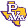Jaypee University of Engineering and Technology

#### (Si10-068) Performance Analysis Of Cosine Window Function, Vikas Misra, Narendra Singh, M. Shukla

##### Applications and Applied Mathematics: An International Journal (AAM)

This paper reviews the mathematical functions called the window functions which are employed in the Finite Impulse Response (FIR) filter design applications as well as spectral analysis for the detection of weak signals. The characteristic properties of the window functions are analyzed and parameters are compared among the known conventional cosine window (CW) functions (Rectangular, Hamming, Hanning, and Blackman) and the variable Kaiser window function. The window function expressed in the time domain can be transformed into the frequency domain by taking the Discrete Fourier Transform (DFT) of the time domain window function. The frequency response of the window function …

2022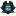East Tennessee State University

#### Bbt Acoustic Alternative Top Bracing Cadd Data Set-Norev-2022jun28, Bill Hemphill

##### STEM Guitar Project’s BBT Acoustic Kit

This electronic document file set consists of an overview presentation (PDF-formatted) file and companion video (MP4) and CADD files (DWG & DXF) for laser cutting the ETSU-developed alternate top bracing designs and marking templates for the STEM Guitar Project’s BBT (OM-sized) standard acoustic guitar kit. The three (3) alternative BBT top bracing designs in this release are
(a) a one-piece base for the standard kit's (Martin-style) bracing,
(c) an X-braced fan-style bracing similar to traditional European or so-called 'classical' acoustic guitars.

The CADD data set for each of the three (3) top bracing designs includes …

Commutative C*-Algebras Generated By Toeplitz Operators On The Fock Space, 2022Louisiana State University and Agricultural and Mechanical College

#### Commutative C*-Algebras Generated By Toeplitz Operators On The Fock Space, Vishwa Nirmika Dewage

##### LSU Doctoral Dissertations

The Fock space $\mathcal{F}(\mathbb{C}^n)$ is the space of holomorphic functions on $\mathbb{C}^n$ that are square-integrable with respect to the Gaussian measure on $\mathbb{C}^n$. This space plays an essential role in several subfields of analysis and representation theory. In particular, it has for a long time been a model to study Toeplitz operators. Grudsky and Vasilevski showed in 2002 that radial Toeplitz operators on $\mathcal{F}(\mathbb{C})$ generate a commutative $C^*$-algebra $\mathcal{T}^G$, while Esmeral and Maximenko showed that $C^*$-algebra $\mathcal{T}^G$ is isometrically isomorphic to the $C^*$-algebra $C_{b,u}(\mathbb{N}_0,\rho_1)$. In this thesis, we extend the result to $k$-quasi-radial symbols acting on the Fock space $\mathcal{F}(\mathbb{C}^n)$. …

Diederich-Fornæss Index On Boundaries Containing Crescents, 2022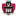University of Arkansas, Fayetteville

#### Diederich-Fornæss Index On Boundaries Containing Crescents, Jason Demoulpied

The worm domain developed by Diederich and Fornæss is a classic example of a boundedpseudoconvex domains that fails to satisfy global regularity of the Bergman Projection, due to the set of weakly pseudoconvex points that form an annulus in its boundary. We instead examine a bounded pseudoconvex domain Ω ⊂ C2 whose set of weakly pseudoconvex points form a crescent in its boundary. In 2019, Harrington had shown that these types of domains satisfy global regularity of the Bergman Projection based on the existence of good vector fields. In this thesis we study the Regularized Diederich-Fornæss index of these domains, …

2021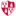Montclair State University

#### Contributions To The Teaching And Learning Of Fluid Mechanics, Ashwin Vaidya

##### Department of Mathematics Facuty Scholarship and Creative Works

This issue showcases a compilation of papers on fluid mechanics (FM) education, covering different sub topics of the subject. The success of the first volume  prompted us to consider another follow-up special issue on the topic, which has also been very successful in garnering an impressive variety of submissions. As a classical branch of science, the beauty and complexity of fluid dynamics cannot be overemphasized. This is an extremely well-studied subject which has now become a significant component of several major scientific disciplines ranging from aerospace engineering, astrophysics, atmospheric science (including climate modeling), biological and biomedical science …

2021King Abdullah University of Science and Technology

#### Lecture 05: The Convergence Of Big Data And Extreme Computing, David Keyes

##### Mathematical Sciences Spring Lecture Series

As simulation and analytics enter the exascale era, numerical algorithms, particularly implicit solvers that couple vast numbers of degrees of freedom, must span a widening gap between ambitious applications and austere architectures to support them. We present fifteen universals for researchers in scalable solvers: imperatives from computer architecture that scalable solvers must respect, strategies towards achieving them that are currently well established, and additional strategies currently being developed for an effective and efficient exascale software ecosystem. We consider recent generalizations of what it means to “solve” a computational problem, which suggest that we have often been “oversolving” them at the …

2021Georgia Institute of Technology

#### Lecture 10: Preconditioned Iterative Methods For Linear Systems, Edmond Chow

##### Mathematical Sciences Spring Lecture Series

Iterative methods for the solution of linear systems of equations – such as stationary, semi-iterative, and Krylov subspace methods – are classical methods taught in numerical analysis courses, but adapting these methods to run efficiently at large-scale on high-performance computers is challenging and a constantly evolving topic. Preconditioners – necessary to aid the convergence of iterative methods – come in many forms, from algebraic to physics-based, are regularly being developed for linear systems from different classes of problems, and similarly are evolving with high-performance computers. This lecture will cover the background and some recent developments on iterative methods and preconditioning …

Linear Combinations Of Harmonic Univalent Mappings, 2021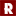California State University, Stanislaus

#### Linear Combinations Of Harmonic Univalent Mappings, Dennis Nguyen

Many properties are known about analytic functions, however the class of harmonic functions which are the sum of an analytic function and the conjugate of an analytic function is less understood. We wish to find conditions such that linear combinations of univalent harmonic functions are univalent. We focus on functions whose image is convex in one direction i.e. each line segment in that direction between points in the image is contained in the image. M. Dorff proved sufficient conditions such that the linear combination of univalent harmonic functions will be univalent on the unit disk. The conditions are: the mappings …

2021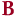Bard College

#### Gibbs Phenomenon For Jacobi Approximations, Riti Bahl

##### Senior Projects Spring 2021

The classical Gibbs phenomenon is a peculiarity that arises when approximating functions near a jump discontinuity with the Fourier series. Namely, the Fourier series "overshoots" (and "undershoots") the discontinuity by approximately 9% of the total jump. This same phenomenon, with the same value of the overshoot, has been shown to occur when approximating jump-discontinuous functions using specific families of orthogonal polynomials. In this paper, we extend these results and prove that the Gibbs phenomenon exists for approximations of functions with interior jump discontinuities with the two-parameter family of Jacobi polynomials Pn(a,b)(x). In particular, we show that for …

2021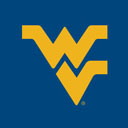West Virginia University

#### Algebraic, Analytic, And Combinatorial Properties Of Power Product Expansions In Two Independent Variables., Mohamed Ammar Elewoday

##### Graduate Theses, Dissertations, and Problem Reports

Let $F(x,y)=I+\hspace{-.3cm}\sum\limits_{\substack{p=1\\m+n=p}}^{\infty}\hspace{-.3cm}A_{m,n}x^my^n$ be a formal power series, where the coefficients $A_{m,n}$ are either all matrices or all scalars. We expand $F(x,y)$ into the formal products $\prod\limits_{\substack{p=1\\m+n=p}}^{\infty}\hspace{-.3cm}(I+G_{m,n}x^m y^n)$, $\prod\limits_{\substack{p=1\\m+n=p}}^{\infty}\hspace{-.3cm}(I-H_{m,n}x^m y^n)^{-1}$, namely the \textit{ power product expansion in two independent variables} and \textit{inverse power product expansion in two independent variables} respectively. By developing new machinery involving the majorizing infinite product, we provide estimates on the domain of absolute convergence of the infinite product via the Taylor series coefficients of $F(x,y)$. This machinery introduces a myriad of "mixed expansions", uncovers various algebraic connections between the $(A_{m,n})$ and the $(G_{m,n})$, and uncovers various algebraic …

Morphology-Dependent Resonances In Two Concentric Spheres With Variable Refractive Index In The Outer Layer: Analytic Solutions, 2021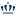Old Dominion University

#### Morphology-Dependent Resonances In Two Concentric Spheres With Variable Refractive Index In The Outer Layer: Analytic Solutions, Umaporn Nuntaplook, John A. Adam

##### Mathematics & Statistics Faculty Publications

In many applications constant or piecewise constant refractive index profiles are used to study the scattering of plane electromagnetic waves by a spherical object. When the structured media has variable refractive indices, this is more of a challenge. In this paper, we investigate the morphology dependent resonances for the scattering of electromagnetic waves from two concentric spheres when the outer shell has a variable refractive index. The resonance analysis is applied to the general solutions of the radial Debye potential for both transverse magnetic and transverse electric modes. Finally, the analytic conditions to determine the resonance locations for this system …

2021Bard College

#### The Complex Propagation Of Light Explained Visually: How To Make A Hologram, Bruno Ray Becher

##### Senior Projects Spring 2021

The complexity of light’s wave nature is shown in the paths that light takes. In this project I will show several useful ways to imagine and predict how light will travel from one place to another. Once light is produced it does not immediately fill a room, instead it undulates through free space as if the space itself was a fluid. Once we understand the way light flows and interacts with its environment not only can we predict and control its shape with a hologram, but also discover clues which give secrets about where the light has been. Telescopes and …

2020Universidad Mayor de San Simón

#### On The Construction And Mathematical Analysis Of The Wavelet Transform And Its Matricial Properties, Diego Sejas Viscarra

We study the properties of computational methods for the Wavelet Transform and its Inverse from the point of view of Linear Algebra. We present a characterization of such methods as matrix products, proving in particular that each iteration corresponds to the multiplication of an adequate unitary matrix. From that point we prove that some important properties of the Continuous Wavelet Transform, such as linearity, distributivity over matrix multiplication, isometry, etc., are inherited by these discrete methods.

This work is divided into four sections. The first section corresponds to the classical theoretical foundation of harmonic analysis with wavelets; it is used …

Perceiving Mathematics And Art, 2020University of Arkansas, Fayetteville

#### Perceiving Mathematics And Art, Edmund Harriss

##### Mic Lectures

Mathematics and art provide powerful lenses to perceive and understand the world, part of an ancient tradition whether it starts in the South Pacific with tapa cloth and wave maps for navigation or in Iceland with knitting patterns and sunstones. Edmund Harriss, an artist and assistant clinical professor of mathematics in the Fulbright College of Arts and Sciences, explores these connections in his Honors College Mic lecture.

2020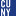The Graduate Center, City University of New York

#### Spectral Sequences For Almost Complex Manifolds, Qian Chen

##### Dissertations, Theses, and Capstone Projects

In recent work, two new cohomologies were introduced for almost complex manifolds: the so-called J-cohomology and N-cohomology [CKT17]. For the case of integrable (complex) structures, the former cohomology was already considered in [DGMS75], and the latter agrees with de Rham cohomology. In this dissertation, using ideas from [CW18], we introduce spectral sequences for these two cohomologies, showing the two cohomologies have natural bigradings. We show the spectral sequence for the J-cohomology converges at the second page whenever the almost complex structure is integrable, and explain how both fit in a natural diagram involving Bott-Chern cohomology and the Frolicher spectral sequence. …

Harmonic Equiangular Tight Frames Comprised Of Regular Simplices, 2020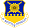Air Force Institute of Technology

#### Harmonic Equiangular Tight Frames Comprised Of Regular Simplices, Matthew C. Fickus, Courtney A. Schmitt

##### Faculty Publications

An equiangular tight frame (ETF) is a sequence of unit-norm vectors in a Euclidean space whose coherence achieves equality in the Welch bound, and thus yields an optimal packing in a projective space. A regular simplex is a simple type of ETF in which the number of vectors is one more than the dimension of the underlying space. More sophisticated examples include harmonic ETFs which equate to difference sets in finite abelian groups. Recently, it was shown that some harmonic ETFs are comprised of regular simplices. In this paper, we continue the investigation into these special harmonic ETFs. We begin …

2020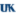University of Kentucky

#### The Direct Scattering Map For The Intermediate Long Wave Equation, Joel Klipfel

##### Theses and Dissertations--Mathematics

In the early 1980's, Kodama, Ablowitz and Satsuma, together with Santini, Ablowitz and Fokas, developed the formal inverse scattering theory of the Intermediate Long Wave (ILW) equation and explored its connections with the Benjamin-Ono (BO) and KdV equations. The ILW equation\begin{align*} u_t + \frac{1}{\delta} u_x + 2 u u_x + Tu_{xx} = 0, \end{align*} models the behavior of long internal gravitational waves in stratified fluids of depth $0< \delta < \infty$, where $T$ is a singular operator which depends on the depth $\delta$. In the limit $\delta \to 0$, the ILW reduces to the Korteweg de Vries (KdV) equation, and in the limit $\delta \to \infty$, the ILW (at least formally) reduces to the Benjamin-Ono (BO) equation.

While the KdV equation is very well understood, a rigorous analysis of inverse scattering for the ILW equation remains to be accomplished. There is currently no rigorous proof that the Inverse Scattering …

Polyphase Equiangular Tight Frames And Abelian Generalized Quadrangles, 2019Air Force Institute of Technology

#### Polyphase Equiangular Tight Frames And Abelian Generalized Quadrangles, Matthew C. Fickus, John Jasper, Dustin G. Mixon, Jesse D. Peterson, Cody E. Watson

##### Faculty Publications

An equiangular tight frame (ETF) is a type of optimal packing of lines in a finite-dimensional Hilbert space. ETFs arise in various applications, such as waveform design for wireless communication, compressed sensing, quantum information theory and algebraic coding theory. In a recent paper, signature matrices of ETFs were constructed from abelian distance regular covers of complete graphs. We extend this work, constructing ETF synthesis operators from abelian generalized quadrangles, and vice versa. This produces a new infinite family of complex ETFs as well as a new proof of the existence of certain generalized quadrangles. This work involves designing matrices whose …

Fluids In Music: The Mathematics Of Pan’S Flutes, 2019Montclair State University

#### Fluids In Music: The Mathematics Of Pan’S Flutes, Bogdan Nita, Sajan Ramanathan

##### Department of Mathematics Facuty Scholarship and Creative Works

We discuss the mathematics behind the Pan’s flute. We analyze how the sound is created, the relationship between the notes that the pipes produce, their frequencies and the length of the pipes. We find an equation which models the curve that appears at the bottom of any Pan’s flute due to the different pipe lengths.

A Course In Harmonic Analysis, 2019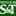Missouri University of Science and Technology

#### A Course In Harmonic Analysis, Jason Murphy

##### AOER Course Materials

These notes were written to accompany the courses Math 6461 and Math 6462 (Harmonic Analysis I and II) at Missouri University of Science & Technology during the 2018-2019 academic year. The goal of these notes is to provide an introduction to a range of topics and techniques in harmonic analysis, covering material that is interesting not only to students of pure mathematics, but also to those interested in applications in computer science, engineering, physics, and so on.